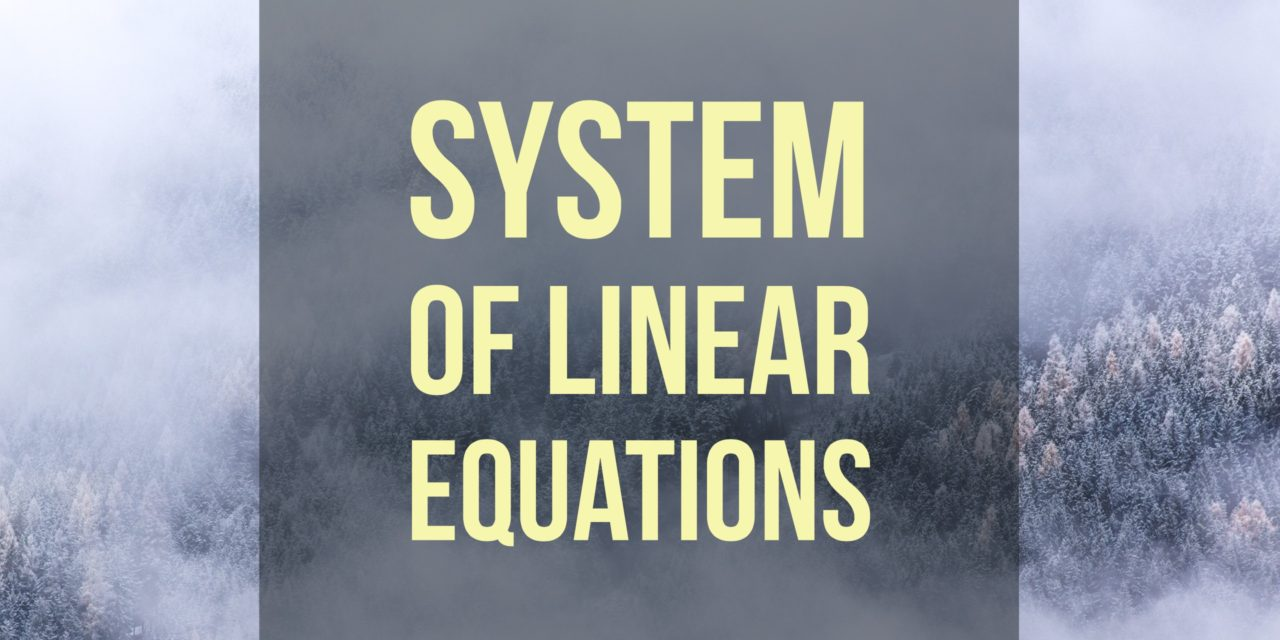# Determine a 2-Digit Number Satisfying Two Conditions## Problem 649

A 2-digit number has two properties: The digits sum to 11, and if the number is written with digits reversed, and subtracted from the original number, the result is 45.

Find the number.Add to solve later

## Solution.

The key to this problem is noticing that our 2-digit number can be written as $10A + B$, where $A, B$ are the 10s and 1s digit respectively.

The digits summing to 11 then yields the equality $A + B = 11$.

The number with digits reversed is $10B + A$, and so the second property yields the equation $10A + B – (10B + A) = 45$. Simplifying, we have the system of equations
\begin{align*}
A+B &= 11\\
9A-9B &= 45
\end{align*}

To solve this system, we create the augmented matrix and use elementary row operations to put it into reduced row-echelon form:
\begin{align*}
\left[\begin{array}{rr|r} 1 & 1 & 11 \\ 9 & -9 & 45 \end{array} \right] \xrightarrow{ R_2 – 9 R_1 } \left[\begin{array}{rr|r} 1 & 1 & 11 \\ 0 & -18 & -54 \end{array} \right]\\[6pt] \xrightarrow{ \frac{-1}{18} R_2 } \left[\begin{array}{rr|r} 1 & 1 & 11 \\ 0 & 1 & 3 \end{array} \right] \xrightarrow{R_1 – R_2} \left[\begin{array}{rr|r} 1 & 0 & 8 \\ 0 & 1 & 3 \end{array} \right].
\end{align*}
With this final augmented matrix, we can read off the solution $A = 8$ and $B = 3$.

Thus our number is $10A + B = 83$.Add to solve later

### More from my site

#### You may also like...

This site uses Akismet to reduce spam. Learn how your comment data is processed.

###### More in Linear Algebra##### Determine Whether Matrices are in Reduced Row Echelon Form, and Find Solutions of Systems

Determine whether the following augmented matrices are in reduced row echelon form, and calculate the solution sets of their associated...

Close